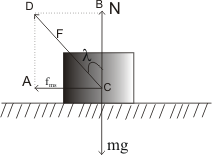# Angle of Friction

## (5) Angle of friction

• It is the angle which the normal force N makes with the contact force when the equilibrium is limiting i.e when the condition of maximum Static friction is reached
• Consider a block of mass "m" resting on a horizontal surface .Weight W=mg of this block is balanced by the normal force (N) of reaction as shown below in the figure.Mathematically
W=mg=-N
• if F is the contact force which each body exerts on the another body then this contact force can be resolved into two components
• Perpendicular Components which is normal force N
• Components parallel to the contact surface known as friction which is usually denoted by the fs,fk or fms
• Angle BCD represented by λ is angle of friction
Since N and fms are components of the contact force F,so we can write
Fcosλ=N
and Fsinλ=fms
or we can say
tanλ=fms/N
Also from the definition of static friction we know that
fmssN So tan λ=μs
Thus we can conclude that coefficient of static friction is equal to the tangent of the angle of friction